#### Chapter 21 Surface Area and Volume of a Sphere R.D. Sharma Solutions for Class 9th MCQ's

Multiple Choice Questions

1. In a sphere the number of faces is
(a) 1
(b) 2
(c) 3
(d) 4

Solution

The number of faces is 1.

2. The total surface area of hemisphere of radius r.
(a) πr2
(b) 2πr2
(c) 3πr2
(d) 4πr2

Solution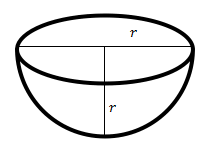So, here we have to find total surface area, which is sum of curved surface area and top area. So total surface area is 2πr2 + πr2 = 3πr2

3. The ratio of the total surface area of a sphere and a hemisphere of same radius is
(a) 2:1
(b) 3:2
(c) 4:1
(d) 4:3

Solution

Total Surface Area of sphere = 4πr2
Total Surface Area of hemisphere = 3πr2
The Ratio is (4πr2)/(3πr2) = 4/3
So, option (d) is correct

4. A Sphere and a cube are of the same height. The ratio of their volumes is
(a) 3:4
(b) 21:11
(c) 4:3
(d) 11:21

Solution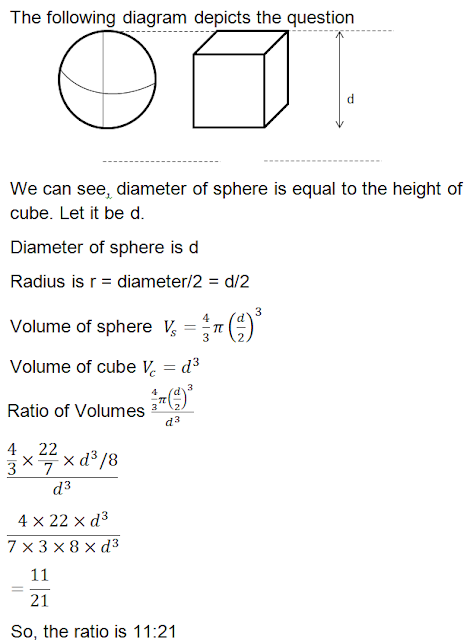5. The largest sphere is cut off from a cube of side 6 cm. The volume of the sphere will be
(a) 27π cm3
(b) 36π cm3
(c) 108 cm3
(d) 12π cm3

Solution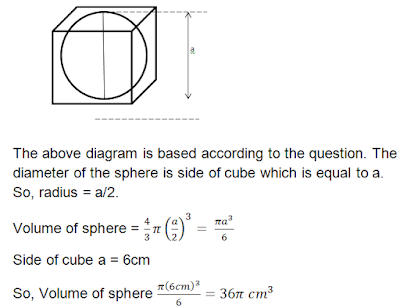6. A cylindrical rod whose height is 8 times of its radius is melted and recast into spherical balls of same radius. The number of balls will be
(a) 4
(b) 3
(c) 6
(d) 8

Solution7. If the ratio of volumes of two sphere is 1:8, then ratio of their surface area is
(a) 1:2
(b) 1:4
(c) 1:8
(d) 1:16

Solution8. If the surface area of a sphere is 144π m2, then its volume in (m3) is
(a) 288π
(b) 316 π
(c) 300π
(d) 188π

Solution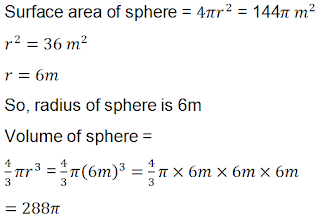9. If a solid sphere of radius 10 cm is moulded into 8 spherical solid balls of equal radius, then the surface area of each ball (in sq.cm) is
(a) 100
(b) 75π
(c) 60π
(d) 50π

Solution10. The ratio between volume of a sphere and volume of circumscribing right circular cylinder is
(a) 2:1
(b) 1:1
(c) 2:3
(d) 1:2

Solution11. If a sphere is inscribed in a cube, then the ratio of the volume of the sphere to the volume of cube is
(a) π :2
(b) π:3
(c) π:4
(d) π:6

Solution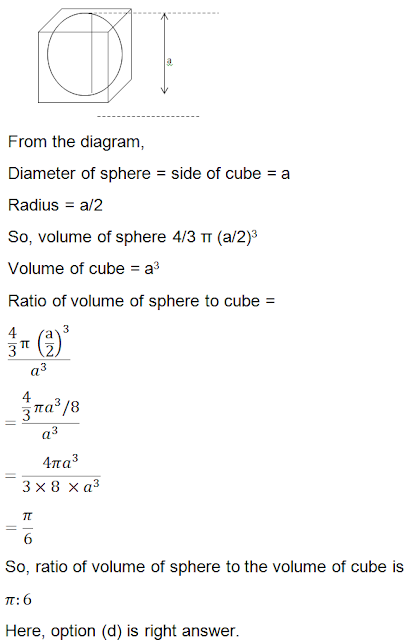12. If a solid sphere of radius r is melted and cast into the shape of a solid cone of height r, then the radius of the base of the cone
(a) 2r
(b) 3r
(c) r
(d) 4r

Solution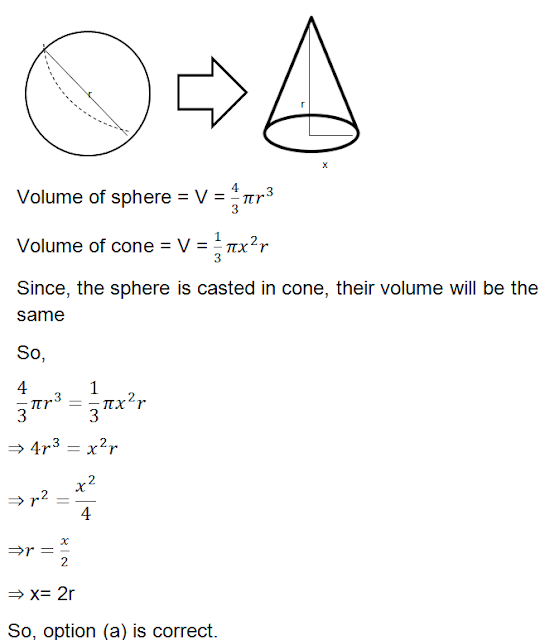13. A sphere is placed inside a right circular cylinder so as to touch the top, base and lateral surface of the cylinder. If the radius of sphere is r, the volume of cylinder is
(a) 4πr3
(b) 8/3 πr3
(c) 2πr3
(d) 8πr3

Solution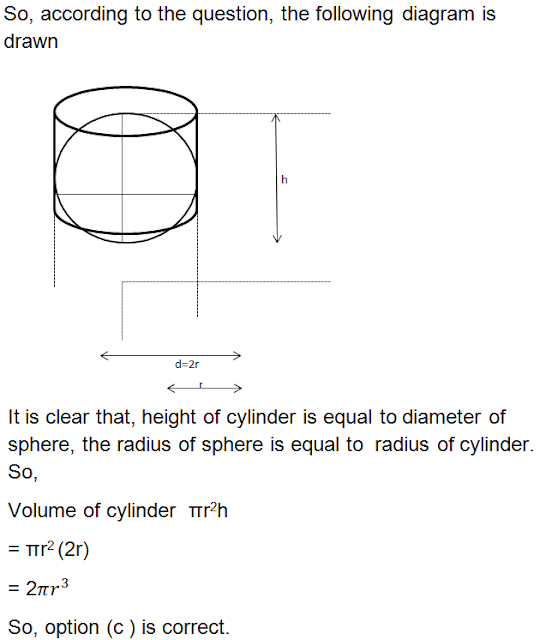14. A cone and a hemisphere have equal bases and equal volumes, the ratio of their heights is
(a) 1:2
(b) 2:1
(c) 4:1
(d) √2: 1

Solution15. A cone and a hemisphere and a cylinder stand on the equal bases and have the same height . The ratio of their volumes is
(a)1:2:3
(b) 2:1:3
(c) 2:3:1
(d) 3:2:1

Solution